# Cramer's rule

Cramer's rule is a way of solving a system of linear equations using determinants.

Suppose we are trying to solve a system of linear equations such that...or Ax = b in matrix form, where

Cramer's Rule says thatwhere Ai is a new matrix formed by replacing the ith column of A with the b vector.

Example

In this case,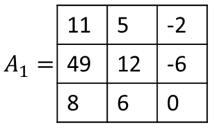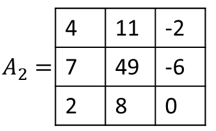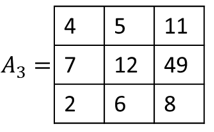By Cramer's rule,

 x1 === -5 x2 === 3 x3 === -8

We can then check that

### Proof

We reinterpret the matrix-vector equation Ax = b as

In other words, b = x1v1 + ... + xnvn where each vi is the ith column of matrix A (see Matrix Multiplication). If we plug this expression for b into Ai, the matrix made by replacing the ith column of A with b, we get:

From the, properties of determinants, we can perform column operations of the type (i) + k(j) → (i) without changing the determinant. Therefore we can use the columns containing v1,..., vi - 1, vi + 1,... vn to subtract out every term in x1v1 + ... + xnvn except for xivi. In other words,

From another property of determinants, a column of type k(i) → (i) has the same effect of multiplying the determinant by k. Therefore we can pull the scalar fact xi from the iith column which contains x1vi. In other words,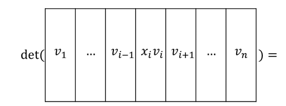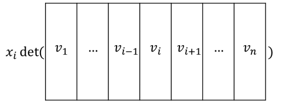Since

combining (1.) and (2.) gives us det(Ai) = xidet(A). Dividing by det(A) gives us, which is the original statement of Cramer's rule.

### Limitations of Cramer's rule

• Because we are dividing by det(A) to get, Cramer's rule only works if det(A) ≠ 0. If det(A) = 0, Cramer's rule cannot be used because a unique solution doesnt exist since there would be infinitely many solutions, or no solution at all.
• Cramer's rule is slow because we have to evaluate a determinant for each xi. When we evaluate each det(Ai) we have to perform Gaussian elimination on each Ai for a total of n times. In comparison, if we were to use the augmented matrix, [A|b], we would only need to perform Gaussian elimination once to solve Ax = b.